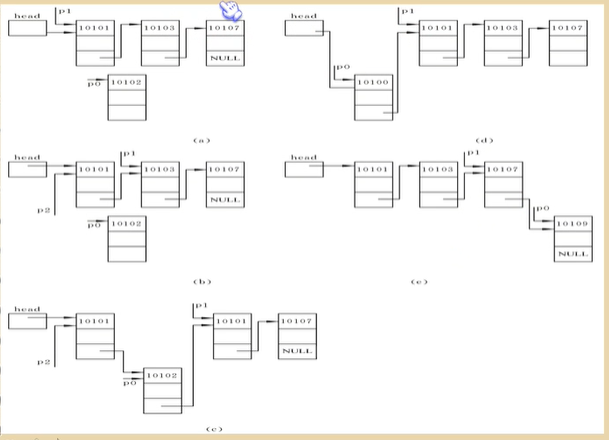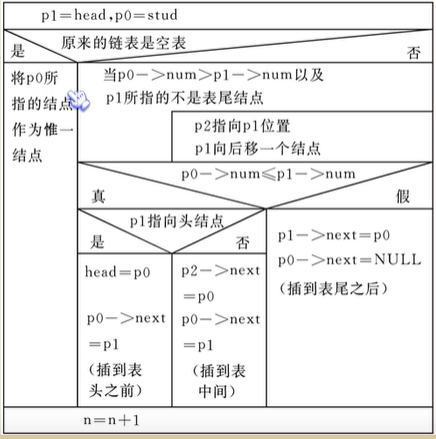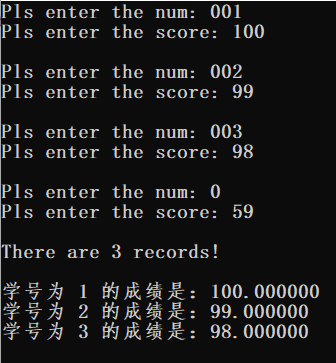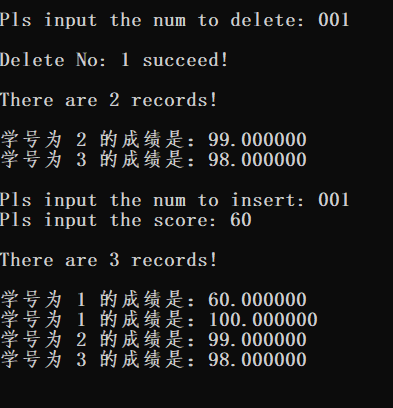## 链表的插入操作_Joaquin233的博客-程序员宅基地

①怎样找到插入的位置；
②怎样实现插入。``````#include <stdio.h>
#include <malloc.h>
#include <stdlib.h>

#define LEN sizeof(struct student)//student结构的大小

struct student *creat();//创建链表
//的头指针，num是要删除的结点num。

struct student *insert (struct student *head,struct student *stu_2);//第一个参数需要被插入的链表
//第二个参数待插入的结构的地址

struct student
{

int num;
float score;
struct student *next;
};

int n;//全局变量，用来记录存放了多少数据。

void main()
{

struct student *stu,*p,stu_2;
int n;

p = stu;
print(p);//打印输出

printf("\nPls input the num to delete: ");
scanf("%d",&n);

printf("\nPls input the num to insert: ");
scanf("%d",&stu_2.num);
printf("Pls input the score: ");
scanf("%f",&stu_2.score);

print(p);

printf("\n\n");
system("pause");
}

struct student *creat()//把数据调进仓库里面
{

struct student *p1,*p2;

p1 = p2 = (struct student *)malloc(LEN);//LEN是student结构的大小

printf("Pls enter the num: ");
scanf("%d",&p1->num);
printf("Pls enter the score: ");
scanf("%f",&p1->score);

n = 0;

while(p1->num)
{

n++;
if(1 == n)
{

}
else
{

p2->next = p1;
}

p2 = p1;
p1 = (struct student *)malloc(LEN);//->
//新建一个student数据结构的对象，为其分配student结构所占用的内存空间。
//sizeof(struct student）为求该对象在内存中占用多少内存空间，然后用malloc函数分配同样大小的空间。
//将指针p1指向该对象，即新分配出的空间。

printf("\nPls enter the num: ");
scanf("%d",&p1->num);
printf("Pls enter the score: ");
scanf("%f",&p1->score);
}

p2->next = NULL;

}

{

struct student *p;
printf("\nThere are %d records!\n\n",n);

{

do
{

printf("学号为 %d 的成绩是：%f\n",p->num,p->score);
p = p->next;//直接用p顺延下去
}while(p);//倘若p的next为NULL，既p=0，结束循环
}
}

struct student *del(struct student *head ,int num)
{

struct student *p1,*p2;

{

printf("\nThis list is NULL\n");
goto end;
}

while(p1->num != num && p1->next != NULL)//如果第一个结点的学号不是要删除的学号，而且存在第二个结点
//则使p2指向p1，再让p1顺延下去，直到找到要删除的数据为止。
{

p2 = p1;
p1 = p1->next;
}
if(num == p1->num)
{

{

}
else                //一般情况：如果要删除的数据不是头结点的数据
{

p2->next = p1->next;//跳过p1当前指向的数据，直接让p2的next指向p1的next
}

printf("\nDelete No: %d succeed!\n",num);
n = n-1;//n是作为一个全局变量，用来记录链表的数据数。
}
else
{

printf("%d not been found!\n",num);
}

end:
}

struct student *insert(struct student *head,struct student *stu_2)
{

struct student *p0,*p1,*p2;

p0=stu_2;
{

p0->next = NULL;
}
else          //如果不是空表的话
{

while((p0->num > p1->num) && (p1->next != NULL))//两种情况退出while，如果是要插入的
//元素p0比链表中任何一个元素都要大，既
//要插到表尾，则退出while，因为此时p1的
//next是空指针NULL。
{

p2=p1;
p1=p1->next;
}

if(p0->num <= p1->num)
{

{

}
else            //普通情况，插入中间
{

p2->next=p0;//在p1指向了p1的next以后，p2的next要指向新插入的元素
}

p0->next = p1;
}
else                //p0->num > p1->num，既p0的num最大，插入到末尾
{

p1->next = p0;
p0->next = NULL;
}
}

n=n+1;                 //由于插入了，所以增加了一位数据成员到链表中

}
``````### 航电oj：Quicksum_不玩游戏的休比的博客-程序员宅基地

**航电oj：Quicksum**#题目描述#输入一个字符串 按照规则一一将字符化为数字再相加 输出总值#空格也是一个字符 数字表示为0#知识点字符串#代码#include<cstdio>#include<cstring>#include<algorithm>#include<iostream>using namespace std;char str;int main(){ while(gets(str))

### mysql distinct 失效_DISTINCT关键字在mysql查询中不起作用-程序员宅基地

MySQL DISTINCT多列您可以将DISTINCT子句与多个列一起使用.在这种情况下,MySQL使用所有列的组合来确定结果集中行的唯一性.如果要解决问题,可以使用group_concat()函数,然后对所有联合查询按catnam进行分组：SELECT name ,GROUP_CONCAT(id)FROM your_tableGROUP BY name ;因此您的查询可以是：SELECT di..._mysql distinct 无效